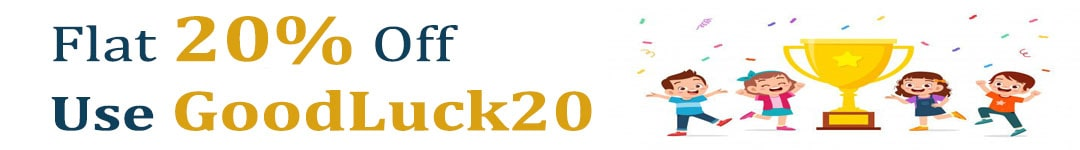International Maths Olympiad Forum By SOF Olympiad Trainer - Page 629

# User ForumSubject :IMO    Class : Class 7

## Ans 1: (Master Answer)

Class : Class 1
The correct answer is B.

Subject :IMO    Class : Class 7

## Ans 1: (Master Answer)

Class : Class 1
Find the LCM of the numbers and add 8 to it.

Subject :IMO    Class : Class 5

## Ans 1:

Class : Class 6
The triangle is not equilateraltriangle. And options 1 and option 2 are also false as triangle donot have 4 straight sides and 4 corners. So answer is option d

Subject :IMO    Class : Class 5

## Ans 1:

Class : Class 8
D. Celsius is warmer than fahrenheit .

Subject :IMO    Class : Class 5

Class : Class 5

## Ans 2:

Class : Class 8
D is the correct answer

Subject :IMO    Class : Class 5

## Ans 1:

Class : Class 9
area= 54 x 28 = 1512 sq cm new length=60 cm breadth= 1512 / 60 = 25.2 cm

Class : Class 8

## Ans 3:

Class : Class 8
D

Subject :IMO    Class : Class 5

## Ans 1:

Class : Class 8
A is the correct answer. 5/8 = 62.5%

## Ans 2:

Class : Class 6
62.5%. itna bhi nahin pata lapata

Subject :IMO    Class : Class 5

## Ans 1:

Class : Class 7
First divide the figure into two rectangles. Than find the area of each rectangle.Add the area of both the rectangles and you will get the the answer.The answer is B

## Ans 2:

Class : Class 7
First divide the figure into two rectangles. Than find the area of each rectangle.Add the area of both the rectangles and you will get the the answer.the answer is B

## Ans 3:

Class : Class 8
B

Subject :IMO    Class : Class 7

## Ans 1: (Master Answer)

Class : Class 1
The correct answer is D.